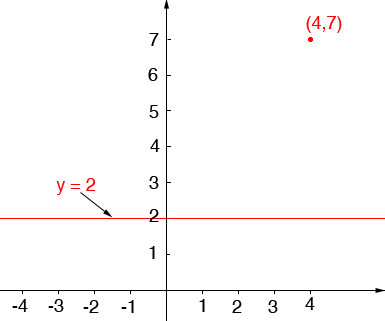SEARCH HOMEMath Central Quandaries & QueriesSubject: parallel and perpendicular lines Name: Chasity Who are you: Student i am suppose to write an equation of a line through the point (4, 7) and parallel to the line y=2. please help meHi Chasity,

The equation y = 2 describes all the points in the plane with y-coordinate 2.This is a horizontal line.Draw the line through (4,7) that is parallel to y = 2. What is the equation of this line?

PennyMath Central is supported by the University of Regina and The Pacific Institute for the Mathematical Sciences.